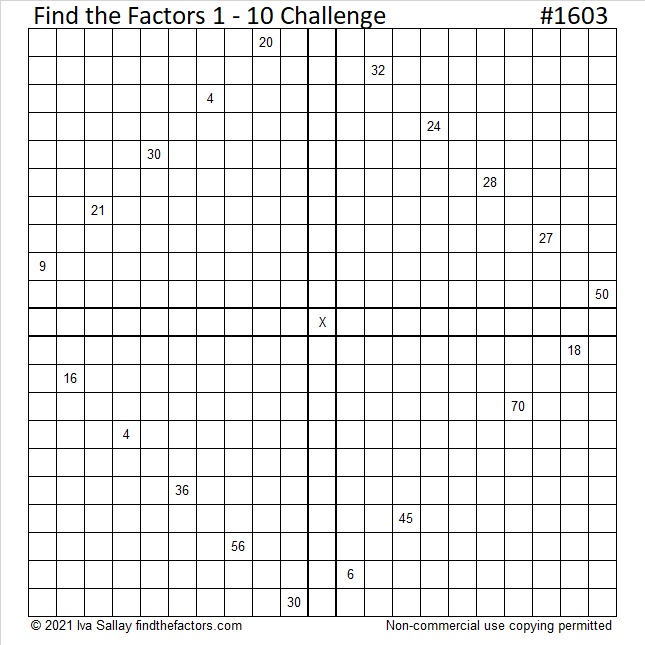# 1603 Challenge Puzzle

### Today’s Puzzle:

Use logic to write the numbers 1 to 10 in each of the four boldly outlined areas so that the puzzle functions like four multiplication tables that work together.Print the puzzles or type the solution in this excel file: 10 Factors 1594-1603.

### Factors of 1603:

• 1603 is a composite number.
• Prime factorization: 1603 = 7 × 229.
• 1603 has no exponents greater than 1 in its prime factorization, so √1603 cannot be simplified.
• The exponents in the prime factorization are 1 and 1. Adding one to each exponent and multiplying we get (1 + 1)(1 + 1) = 2 × 2 = 4. Therefore 1603 has exactly 4 factors.
• The factors of 1603 are outlined with their factor pair partners in the graphic below.### More about the Number 1603:

1603 is the hypotenuse of a Pythagorean triple:
420-1547-1603, which is 7 times (60-221-229).